# Matplotlib基础全攻略

+关注继续查看

Matplotlib是Python中最流行的绘图库，它模仿MATLAB中的绘图风格，提供了一整套与MATLAB相似的绘图API，通过API，我们可以轻松地绘制出高质量的图形。

1、开场例子

ChinaBank = pd.read_csv('data/ChinaBank.csv',index_col = 'Date')
ChinaBank = ChinaBank.iloc[:,1:]
ChinaBank.index = pd.to_datetime(ChinaBank.index)
Close = ChinaBank.Close


plt.plot(Close['2014'])
plt.show()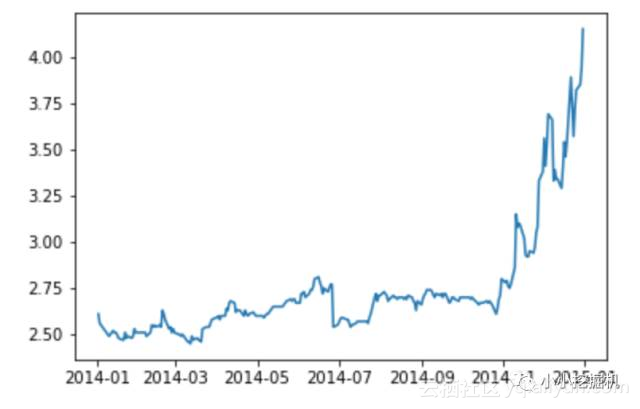2、修改绘图属性

2.1 坐标

plt.plot([1,1,0,0,-1,0,1,1,-1])
plt.show()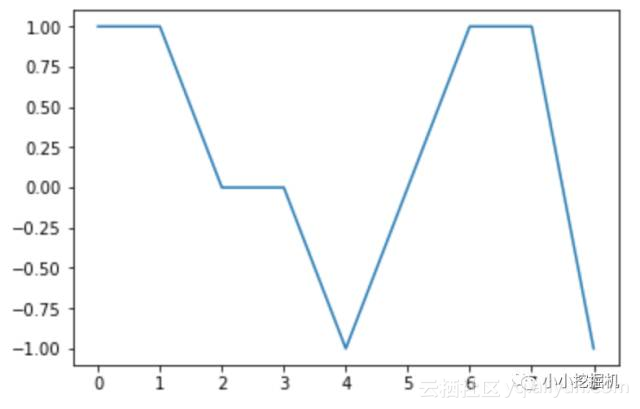plt.plot([1,1,0,0,-1,0,1,1,-1])
plt.ylim(-1.5,1.5)
plt.show()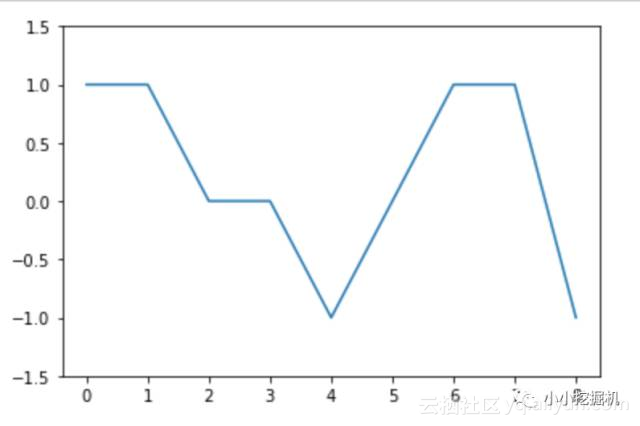location:指坐标的位置.
labels：对应的坐标位置显示的标签.

plt.plot([1,1,0,0,-1,0,1,1,-1])
plt.ylim(-1.5,1.5)
plt.xticks(range(9),['02-01','02-02','02-03','02-04',
'02-05','02-06','02-07','02-08','02-09'],rotation=45)
plt.show()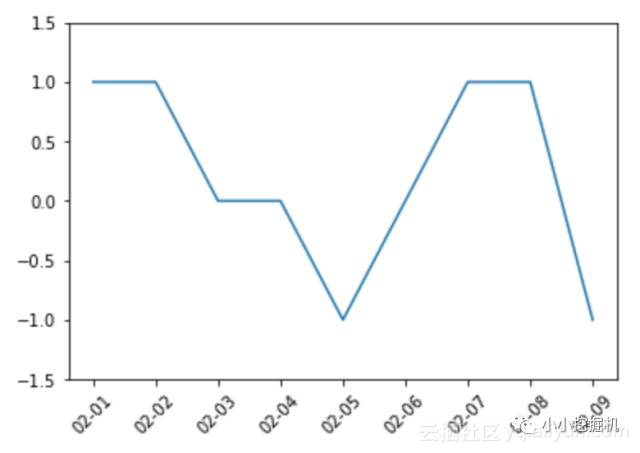2.2 添加文本

plt.plot(Close['2014'])
plt.title('中国银行2014年收盘价曲线',loc='right')
plt.show()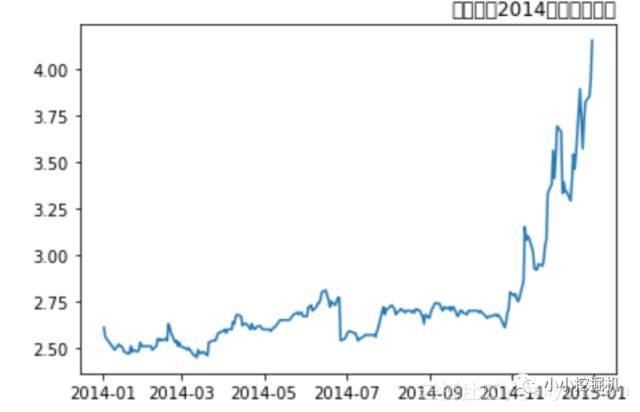from matplotlib.font_manager import FontManager, FontProperties
def getChineseFont():
return FontProperties(fname='/System/Library/Fonts/PingFang.ttc')

plt.plot(Close['2014'])
plt.title('中国银行2014年收盘价曲线',loc='right',fontproperties=getChineseFont())
plt.show()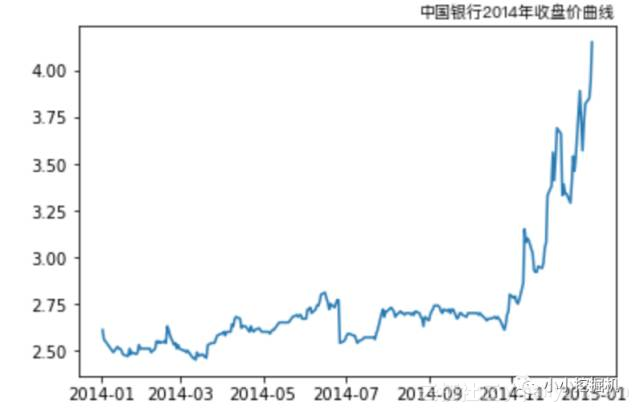plt.plot(Close['2014'])
plt.title('中国银行2014年收盘价曲线',loc='center',fontproperties=getChineseFont())
plt.xlabel('日期',fontproperties=getChineseFont())
plt.ylabel('收盘价',fontproperties=getChineseFont())
plt.show()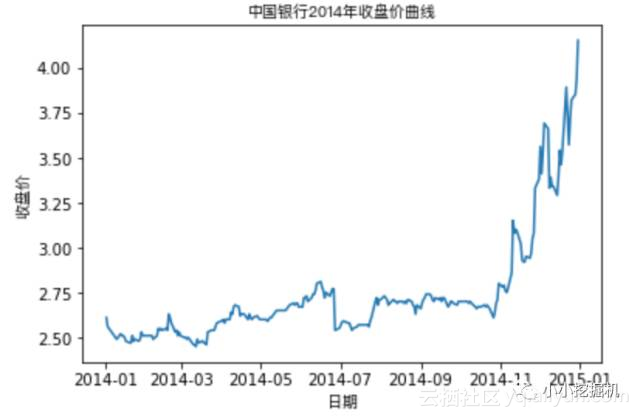plt.plot(Close['2014'])
plt.title('中国银行2014年收盘价曲线',loc='center',fontproperties=getChineseFont())
plt.xlabel('日期',fontproperties=getChineseFont())
plt.ylabel('收盘价',fontproperties=getChineseFont())
plt.grid(True,axis='y')
plt.show()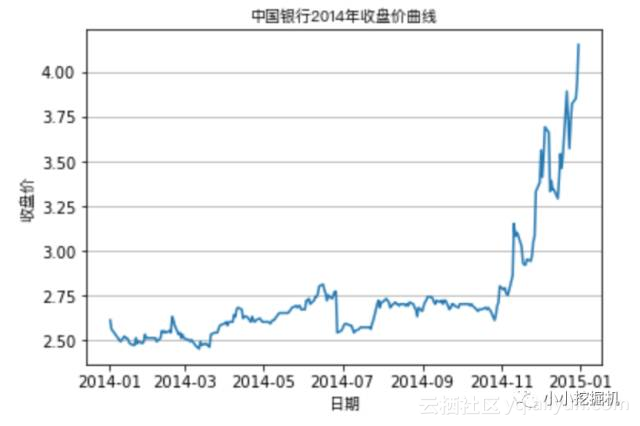Open=ChinaBank.Open
plt.plot(Close['2014'],label='收盘价')
plt.plot(Open['2014'],label='开盘价')
plt.legend(loc='best',prop=getChineseFont())
plt.show()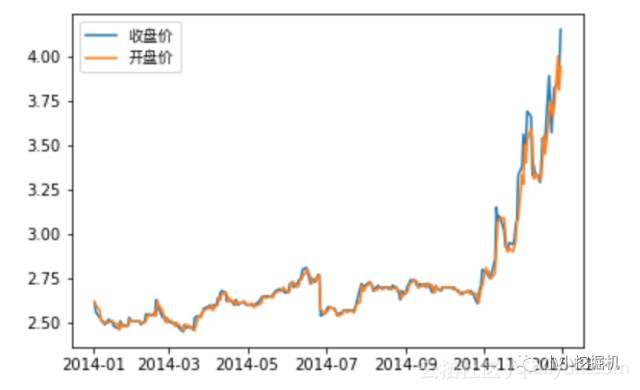2.3 多种线条属性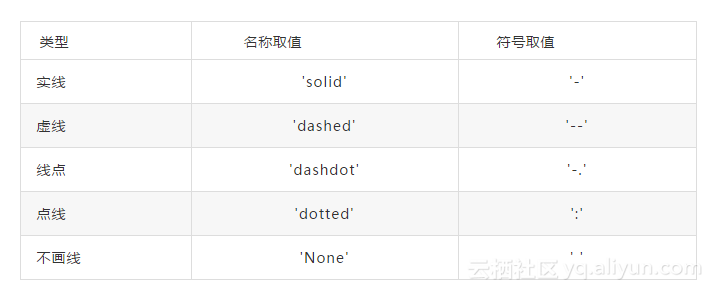plt.plot(Close['2014'],label='收盘价',linestyle='solid')
plt.plot(Open['2014'],label='开盘价',ls='-.')
plt.legend(loc='best',prop=getChineseFont())
plt.xlabel('日期',fontproperties=getChineseFont())
plt.ylabel('收盘价',fontproperties=getChineseFont())
plt.title('中国银行2014年收盘价曲线',loc='center',fontproperties=getChineseFont())
plt.grid(True,axis='y')
plt.show()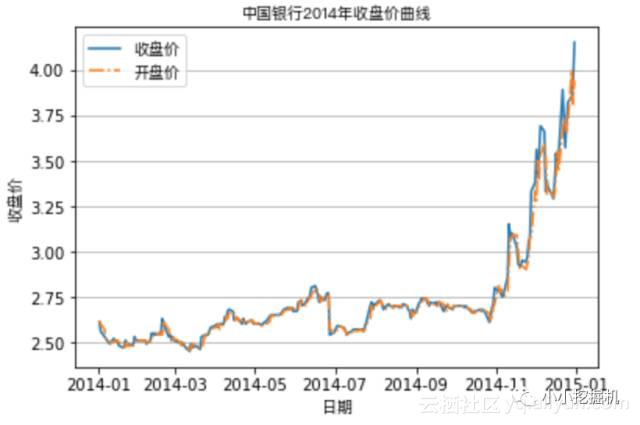plt.plot(Close['2014'],label='收盘价',c='r',linestyle='solid')
plt.plot(Open['2014'],label='开盘价',c='b',ls=':')
plt.legend(loc='best',prop=getChineseFont())
plt.xlabel('日期',fontproperties=getChineseFont())
plt.ylabel('收盘价',fontproperties=getChineseFont())
plt.title('中国银行2014年收盘价曲线',loc='center',fontproperties=getChineseFont())
plt.grid(True,axis='y')
plt.show()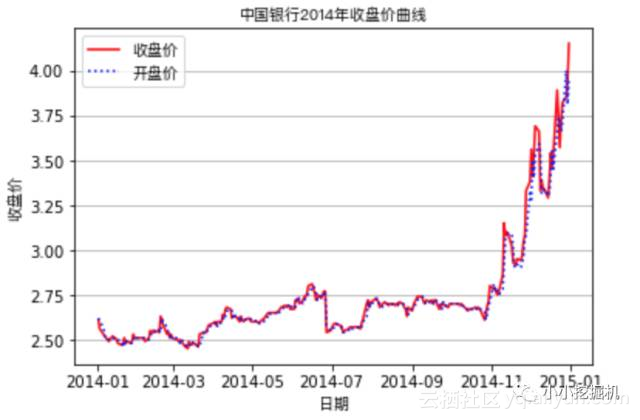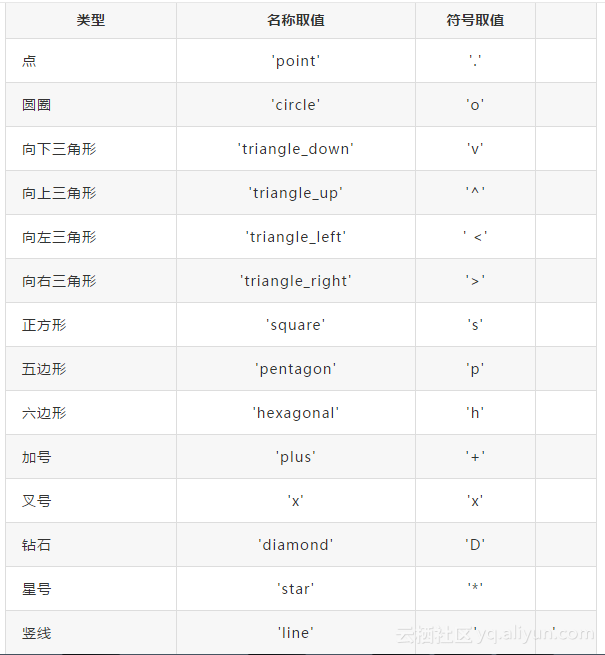plt.plot(Close['2014'],label='收盘价',c='r',marker='o',linestyle='solid')
plt.plot(Open['2014'],label='开盘价',c='b',marker='v',ls='-.')
plt.legend(loc='best',prop=getChineseFont())
plt.xlabel('日期',fontproperties=getChineseFont())
plt.ylabel('收盘价',fontproperties=getChineseFont())
plt.title('中国银行2014年收盘价曲线',loc='center',fontproperties=getChineseFont())
plt.grid(True,axis='y')
plt.show()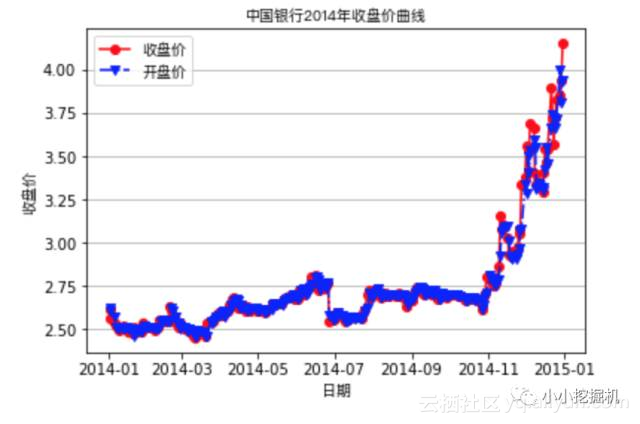plt.plot(Close['2014'],label='收盘价',c='r',marker='o',linestyle='solid',linewidth=1)
plt.plot(Open['2014'],label='开盘价',c='b',marker='>',ls='-.',lw=3)
plt.legend(loc='best',prop=getChineseFont())
plt.xlabel('日期',fontproperties=getChineseFont())
plt.ylabel('收盘价',fontproperties=getChineseFont())
plt.title('中国银行2014年收盘价曲线',loc='center',fontproperties=getChineseFont())
plt.grid(True,axis='y')
plt.show()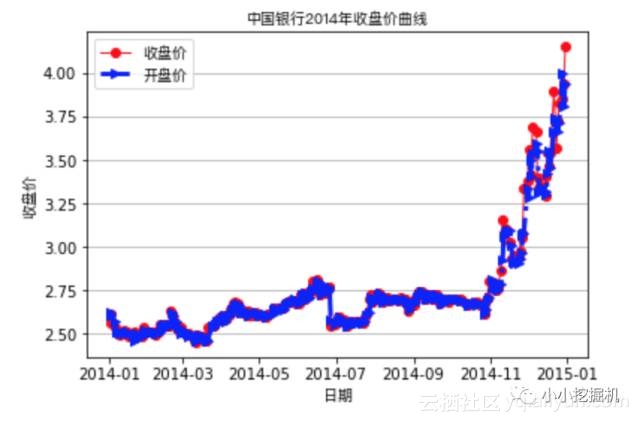plt.plot(Close['2014'],'--rD',label='收盘价',linewidth=1)
plt.plot(Open['2014'],'--b>',label='开盘价',lw=3)
plt.legend(loc='best',prop=getChineseFont())
plt.xlabel('日期',fontproperties=getChineseFont())
plt.ylabel('收盘价',fontproperties=getChineseFont())
plt.title('中国银行2014年收盘价曲线',loc='center',fontproperties=getChineseFont())
plt.grid(True,axis='y')
plt.show()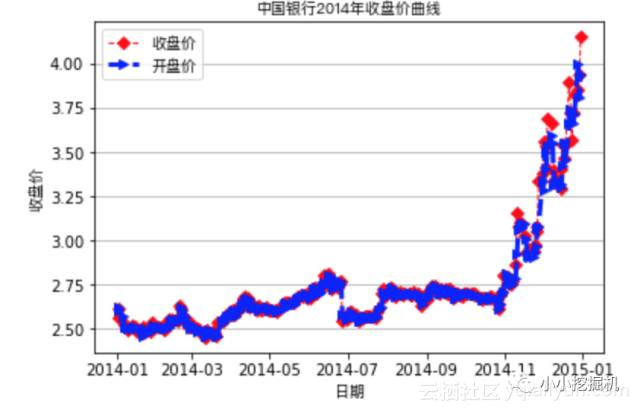3、常见图形绘制

3.1 柱状图

plt.bar(left=[2,3,4,5],height=[228,35,81,1],bottom=2,width=1,color='r',edgecolor='b')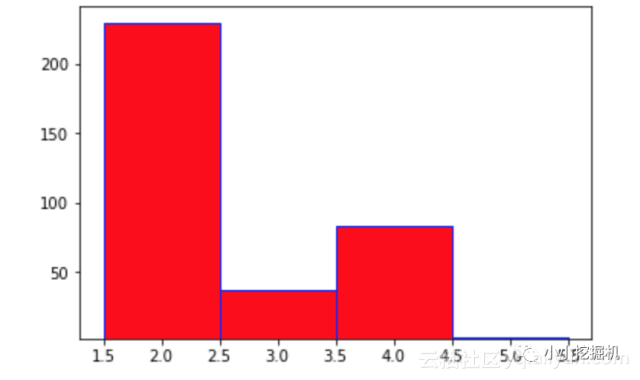plt.barh([2,3,4,5],[228,35,81,1],height=1.0,color='r',edgecolor='b')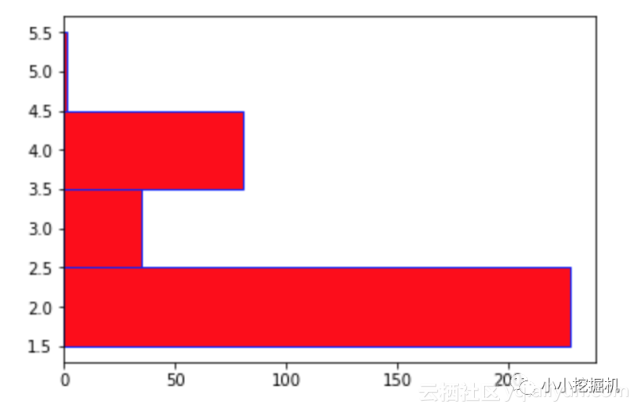3.2 直方图

bins用于设置直方图分布区间的个数;
range用于设置直方图的小矩形的最小值与最大值;
orientation用于设置直方图的水平或者垂直显示，默认是竖直的直方图，可以将orientation设置为horizontal使其变为水平直方图.

plt.hist(Close,bins=12)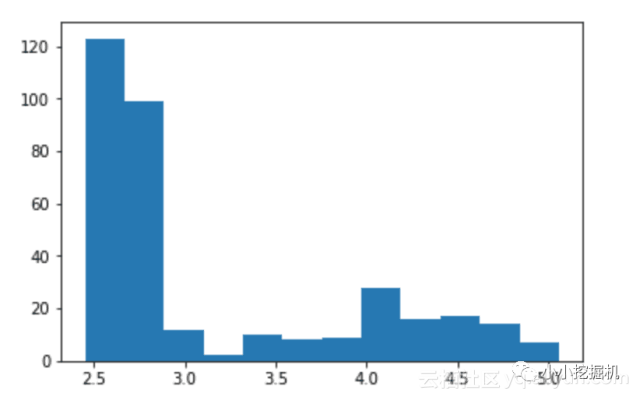plt.hist(Close,bins=12,orientation='horizontal')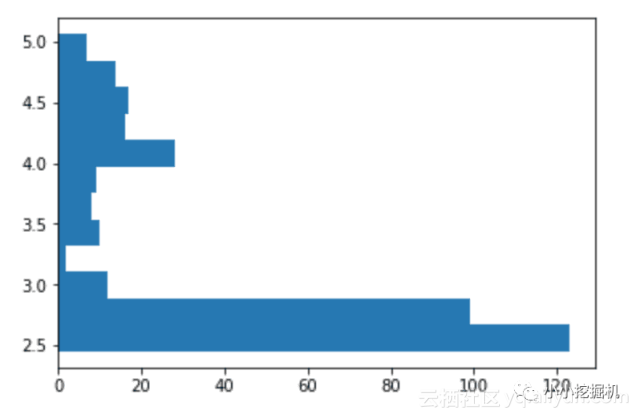plt.hist(Close,range=(2.3,5.5),orientation='vertical',cumulative=True,color='r',edgecolor='b')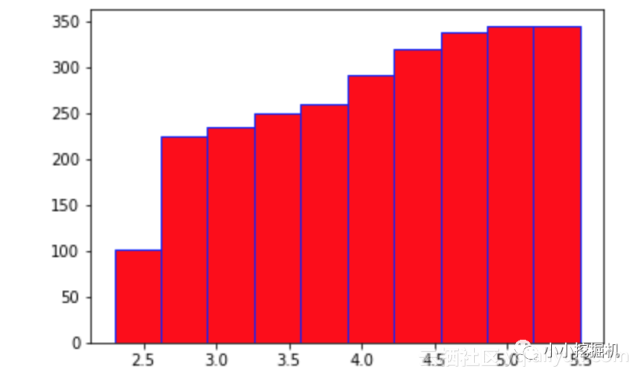3.3 饼图

labels：用于设置扇形图的标签
colors：用于设置扇形图的颜色

plt.pie([228,35,81,1],labels=('(2,3]','(3,4]',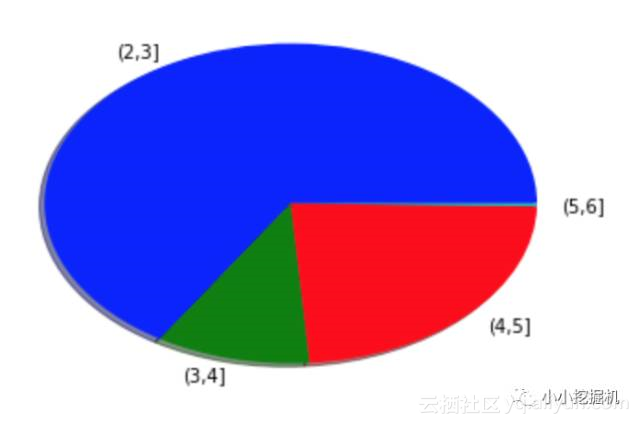3.4 箱线图

pyplot的boxplot函数用于绘制箱线图，主要有以下几个参数:
notch：表示箱线图的类型，默认为False，即绘制矩形箱线图，如果取值为True，表示绘制锯齿状箱线图
labels：表示箱形图的标签，一般为字符串序列类型

import numpy as np
prcData = ChinaBank.iloc[:,:4]
data = np.array(prcData)
plt.boxplot(data,labels=('Open','High','Low','Close'))
plt.title('中国银行股票箱线图',fontproperties=getChineseFont())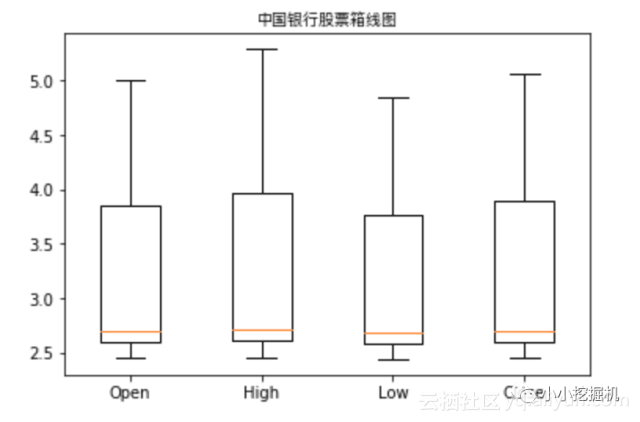4、多图绘制

fig = plt.figure(1)


ax1 = fig.add_axes([0.1,0.1,0.3,0.3])


fig = plt.figure(1)
ax1.plot(Close[:10])
ax1.set_title('前十个交易日收盘价',fontproperties=getChineseFont())
ax1.set_xlabel('日期',fontproperties=getChineseFont())
ax1.set_ylabel('收盘价',fontproperties=getChineseFont())
ax1.set_ylim(2.4,2.65)

ax2.plot(Open[:10])
ax2.set_title('前十个交易日开盘价',fontproperties=getChineseFont())
ax2.set_xlabel('日期',fontproperties=getChineseFont())
ax2.set_ylabel('开盘价',fontproperties=getChineseFont())
ax2.set_ylim(2.4,2.65)
plt.show()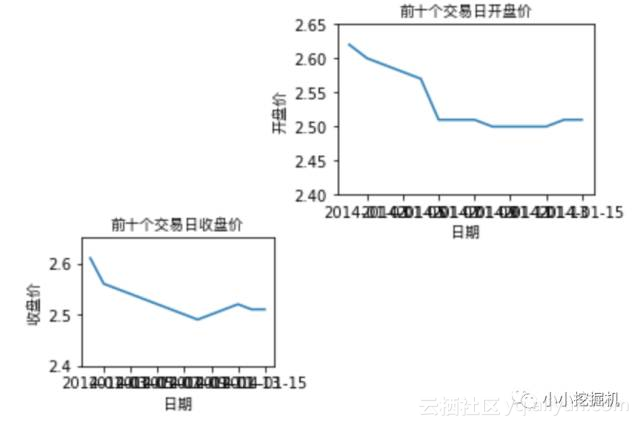fig = plt.figure()
ax1 = plt.subplot(221)
ax2 = plt.subplot(222)
ax3 = plt.subplot(223)
ax4 = plt.subplot(224)
plt.show()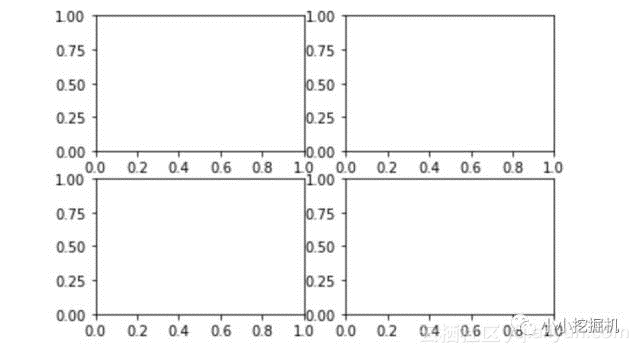Close15 = Close['2015']
ax1 = plt.subplot(221)
ax1.plot(Close15,color='k')
ax1.set_xlabel('日期',fontproperties=getChineseFont())
ax1.set_ylabel('收盘价',fontproperties=getChineseFont())
ax1.set_title('中国银行2015年收盘价曲线',fontproperties=getChineseFont())

Volume15 = ChinaBank['Volume']['2015']

Open15 = Open['2015']
ax2 = plt.subplot(212)

left1 = Volume15.index[Close15>=Open15]
hight1 = Volume15[left1]
ax2.bar(left1,hight1,color='r')
left2 = Volume15.index[Close15<Open15]
hight2 = Volume15[left2]
ax2.bar(left2,hight2,color='g')

ax2.set_ylabel('成交量')
ax2.set_title('中国银行2015年成交量柱状图')

plt.show()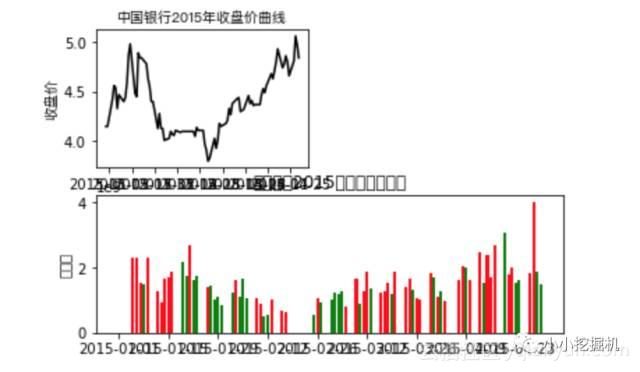《网络安全法》强化关键信息基础设施保护
2147 019348 0Lua 基础
Lua 基础语法及示例
416 0InDesign是一款主流的专业排版设计软件，被广泛应用于书刊、媒体、平面设计、印刷出版和数字媒体等领域。本书图文并茂地讲解中文版InDesign CC 2018的各项功能、应用技巧和设计手段。为了达到学以致用的目的，全书以丰富示例详解软件的各项实用功能和重点参数，并穿插介绍大量的工作流程以及书刊、画册的编排经验和设计方法。另外，本书还提供了几个具有挑战性的综合案例，让你跟随书中的设计思路一步步地实现项目要求的效果。本书提供了丰富的设计案例和供下载的教学视频，特别适合InDesign新手阅读。对具有一定使用经验的用户，其中的案例也有很好的参考价值。本书还可作为职业学校、培训机构的教学用书。
588 029147 01100 0《逻辑与计算机设计基础（原书第5版）》——1.4　算术运算

1284 0《逻辑与计算机设计基础（原书第5版）》——1.7　格雷码

1351 0《逻辑与计算机设计基础（原书第5版）》——1.3　数制

1228 0Java 基础 之 逻辑运算
801 0

7195

9

+ 订阅

JS零基础入门教程（上册）